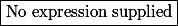# Matematické Fórum

Nevíte-li si rady s jakýmkoliv matematickým problémem, toto místo je pro vás jako dělané.

Nástěnka
! 2.11.2020 (L) Vykreslete si svůj první matematický výraz přes MathJax!
! 04.11.2016 (Jel.) Čtete, prosím, před vložení dotazu, děkuji!
17.01.2016 (Jel.) Rok 2016 s novými a novějšími krystaly od kolegy Pavla!
17.01.2016 (Jel.) Nabídka knih z oborů matematiky, fyziky, chemie
23.10.2013 (Jel.) Zkuste před zadáním dotazu použít některý z online-nástrojů, konzultovat použití můžete v sekci CAS.

Nejste přihlášen(a). Přihlásit

## #1 18. 03. 2020 10:28

stuart clarkPříspěvky: 1007
Reputace:

### Minimum area

Let$g:\mathbb{R}\rightarrow \mathbb{R}$ be a continuous function such that$\int^{1}_{0}g(xt)dt = 0$ for all$x\in \mathbb{R},$

Then minimum area bounded by the curve$y=g(x),y=x^4-4x-k$ and the ordinate$x=2$ to$x=4$ is

Offline

## #2 22. 08. 2020 00:27

BatiPříspěvky: 2290
Reputace:   180

110

Offline